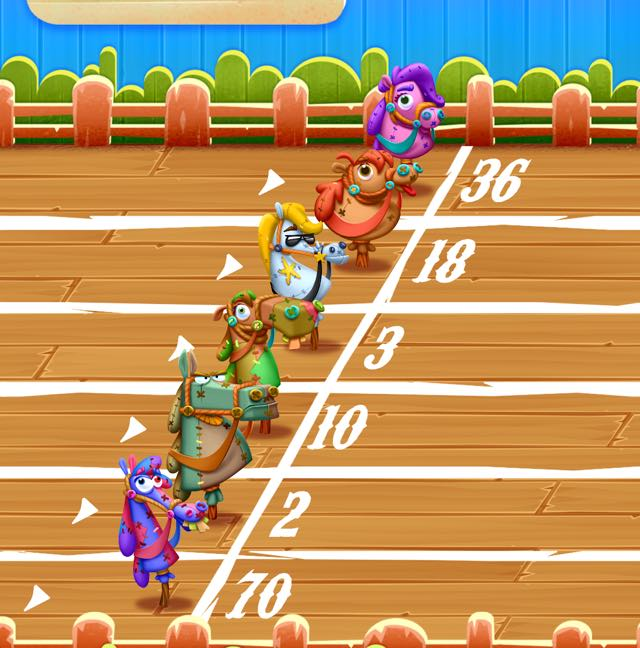DEMO MODEThe game board is divided into 6 parts,each part contains a number (2 , 3, 6, 12, 30, 60). There are also 2 multiplier fields on the board.Players can bet on any of the six numbers. The goal of the game is to guess on which number the ball will stop.
If the ball stops on a number, the spin is finished, and we can calculate the win.
In case the ball stops on a multiplier X, the ball is droped again and the next win is multiplied by that multiplier. Multipliers can be drawn more than once in a row. In that case multipliers are stacked (x2 x5 x2 = x20), and the drawing continues until a number is drawn.

### Win calculator:

part 2 = bet x 2
part 3 = bet x 3
part 6 = bet x 6
part 12 = bet x 12
part 30 = bet x 30
part 60 = bet x 60
multiplier x2 = 2 x next odd
multiplier x5 = 5 x next odd
RTP is 95.24%.0
Balance increases for
0
Balance decreases for
0Connecting...